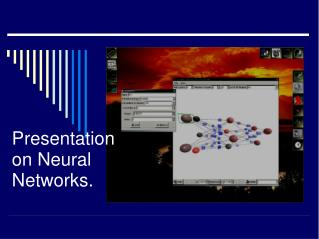DownloadDownload PresentationPresentation on Neural Networks.

# Presentation on Neural Networks.

Télécharger la présentation## Presentation on Neural Networks.

- - - - - - - - - - - - - - - - - - - - - - - - - - - E N D - - - - - - - - - - - - - - - - - - - - - - - - - - -
##### Presentation Transcript

1. Presentation on Neural Networks.

2. Basics Of Neural Networks Neural networks refers to a connectionist model that simulates the biophysical information processing occurring in the nervous system. It can also be defined as an interconnected assembly of simple processing elements ,units or nodes whose functionality is loosely based on the animal neuron. And a cognitive information processing structure based (on models of brain function. In a more formal engineering context a highly parallel dynamical system with the topology of a directed graph that can carry out information processing by means of it's state response to continuous or initial input.

3. Facts 1. Knowledge is acquired by the network from its environment through a learning process. 2. Interneuron connection strengths known as synaptic weights are used to store the acquired knowledge

4. Three components of a neural network A. A set of nodes connected together via links. B. An activation rule that each node follows in updating its activation level. C. An activation function for limiting the amplitude of the output of a neuron.

5. Three basic elements of a neuronal model a. A set of synapses or connecting links each of which is characterized by a weight. b. An adder for summing the input signals. c. An activation function for limiting the amplitude of the output of a neuron.

6. Classification of neural networks Binary valued inputs and continuous valued inputs. Trained with and without supervision. Those with and without adaptive training.

7. Unsupervised learning in unsupervised learning or self organized learning the network is not given • Any external indication as to what the correct responses should be. It simply learns by the environment. • Unsupervised learning aims at finding a certain kind of regularity in the data represented by the exemplars. • In unsupervised learning correlation rule may be applied to calculate weight changes. Supervised learning The adjustment of weights is done according to the desired or correct output available under specific input pattern. Error correction is the most common form of supervised learning. Error is defined as the difference between the direct response and actual response of the network.

8. Single layer perceptrons The single layer perceptron was among the first and simplest learning machines that are trainable.

9. Multi layer preceptrons 1.The model of each neuron in the network includes a non linear activation function. 2. The network contains one or more layers of hidden neurons that are not part of input or output of the network. 3. The network exhibits a high degree of connectivity determined by the synapses of the network.

10. Recurrent Networks • There are two ways of feedback • a. Local feedback at the level of a single neuron inside the network. • b.Global feedback encompassing the whole network Networks Neural networks with one or more feedback loops are referred to as recurrent networks

11. Another way of classifying neural network 1. Multilayer feed forward networks 2. Kohonen self organizing feature maps. 3. Hopfield networks

12. Multilayer perceptron networks These are feed forward nets with one of more layers of nodes between input and output nodes.

13. Kohonen networks and learning vector quantization A simple kohonen net architecture consists of two layers an input layer and a kohonen output layer.

14. Kohonen network operates in two steps . First it selects the unit whose connection weight vector is closest to the current input vector as the winning After a winning neighborhood is selected the connection vectors to the units whose output values are rotated toward the input vector.

15. SOFM and competitive learning The goal of SOFM is the mapping of an input space of n-dimensions into one or two dimensional lattice which comprises the output space such that a meaningful topological ordering exists within the output space. The input layer is connected to the output layer through feed forward connections.

16. Hopfield network • A Hopfield network follows a gradient descent rule. Once it reaches a global minimum is stuck there until some randomness is thrown to make it reach global minimum. • Simulated annealing is a method that introduces randomness to allow system to jump out of global minimum Its a network in which every unit is connected to every other unit and the connections are symmetric. A Hopfield network consists of the following algorithms- 1. Assigning synaptic weights. 2. Initializaion the search items. 3. Activation weight computation and iteration. 4. Convergence.

17. Semantic networks Semantic networks have nodes that represent concepts and connections that represent associations between them. There is some sort of inheritance links between objects and these links are called "IS A" links.